# Rasterization Aaron Bloomfield CS 445 Introduction to Graphics

• Slides: 45
Download presentationRasterization Aaron Bloomfield CS 445: Introduction to Graphics Fall 2006 (Slide set originally by David Luebke)The Rendering Pipeline: A Tour Transform Illuminate Transform Clip Project Rasterize Model & Camera Parameters Rendering Pipeline Framebuffer Display 2After Rasterization, what’s next? n Visible surface determination n Anti-aliasing n (image from here) 3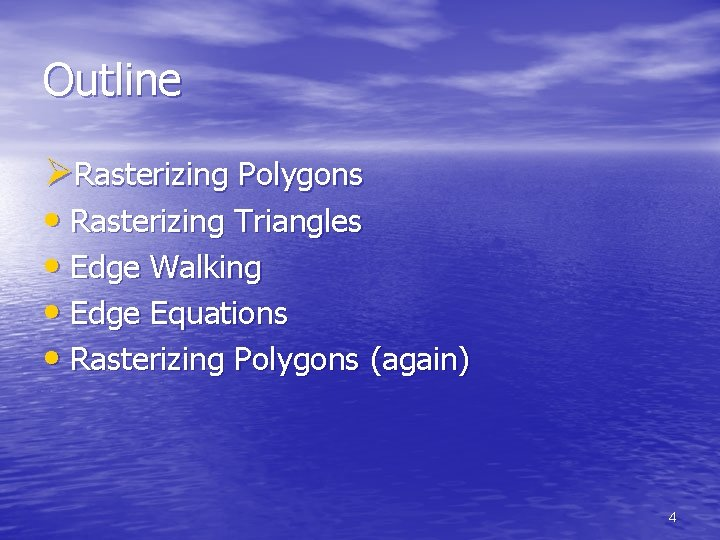Outline ØRasterizing Polygons • Rasterizing Triangles • Edge Walking • Edge Equations • Rasterizing Polygons (again) 4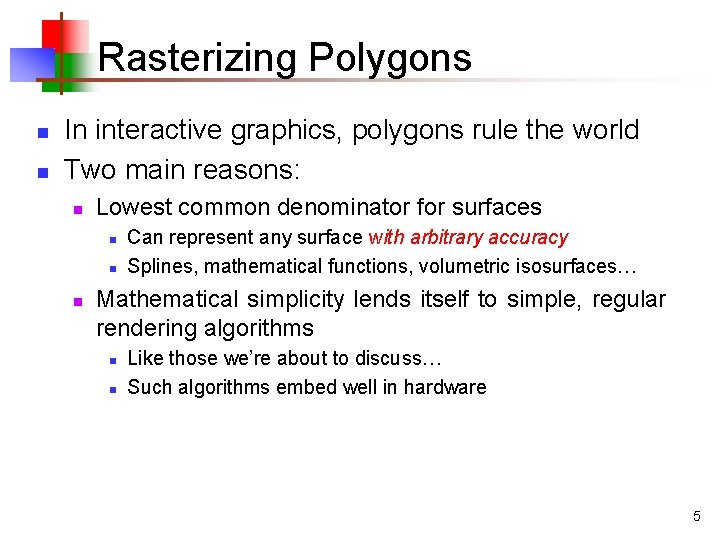Rasterizing Polygons n n In interactive graphics, polygons rule the world Two main reasons: n Lowest common denominator for surfaces n n n Can represent any surface with arbitrary accuracy Splines, mathematical functions, volumetric isosurfaces… Mathematical simplicity lends itself to simple, regular rendering algorithms n n Like those we’re about to discuss… Such algorithms embed well in hardware 5Rasterizing Polygons n Triangle is the minimal unit of a polygon n All polygons can be broken up into triangles n n Triangles are guaranteed to be: n n n Convex, concave, complex Planar Convex What exactly does it mean to be convex? 6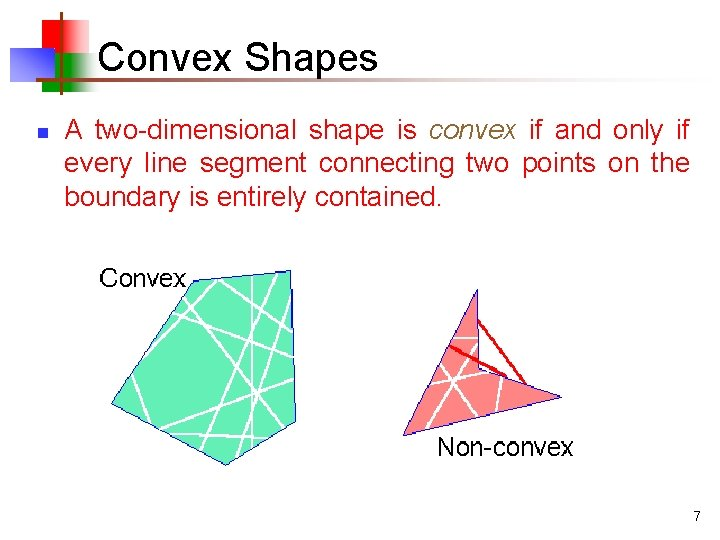Convex Shapes n A two-dimensional shape is convex if and only if every line segment connecting two points on the boundary is entirely contained. 7Convex Shapes n n Why do we want convex shapes for rasterization? One good answer: because any scan line is guaranteed to contain at most one segment or span of a triangle n n Another answer coming up later Note: Can also use an algorithm which handles concave polygons. It is more complex than what we’ll present here! 8Decomposing Polys Into Tris n Any convex polygon can be trivially decomposed into triangles n n Draw it Any concave or complex polygon decomposed into triangles, too n can be Non-trivial! 9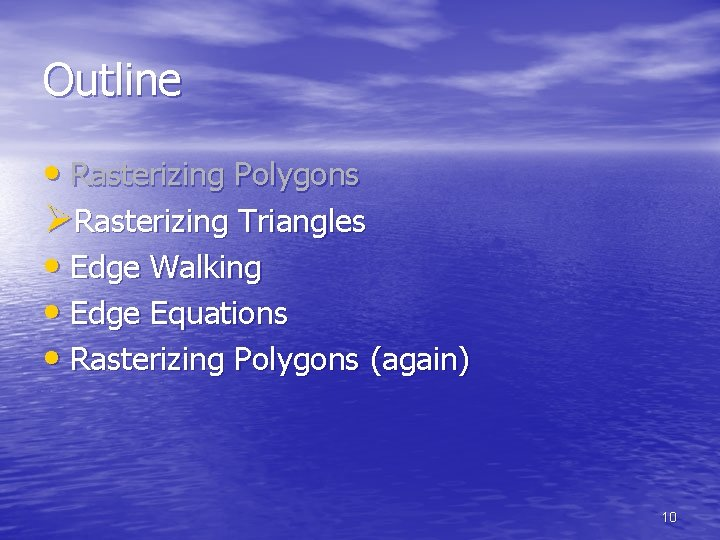Outline • Rasterizing Polygons ØRasterizing Triangles • Edge Walking • Edge Equations • Rasterizing Polygons (again) 10Rasterizing Triangles n n Interactive graphics hardware commonly uses edge walking or edge equation techniques for rasterizing triangles Two techniques we won’t talk about much: n n Recursive subdivision of primitive into micropolygons (REYES, Renderman) Recursive subdivision of screen (Warnock) 11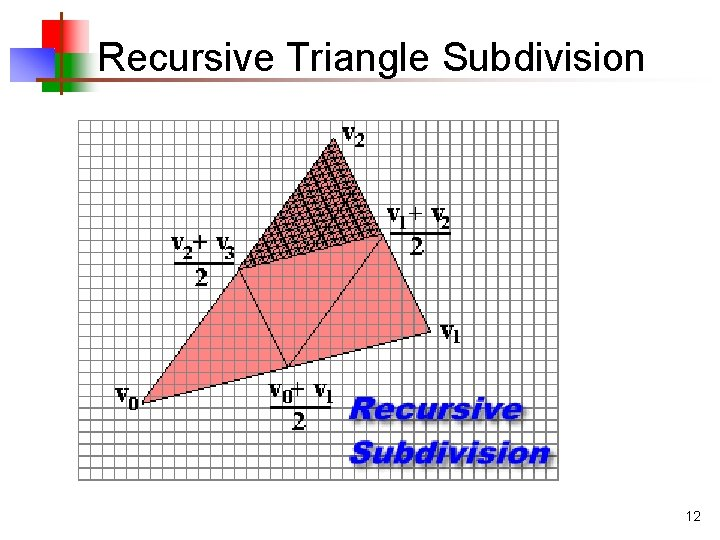Recursive Triangle Subdivision 12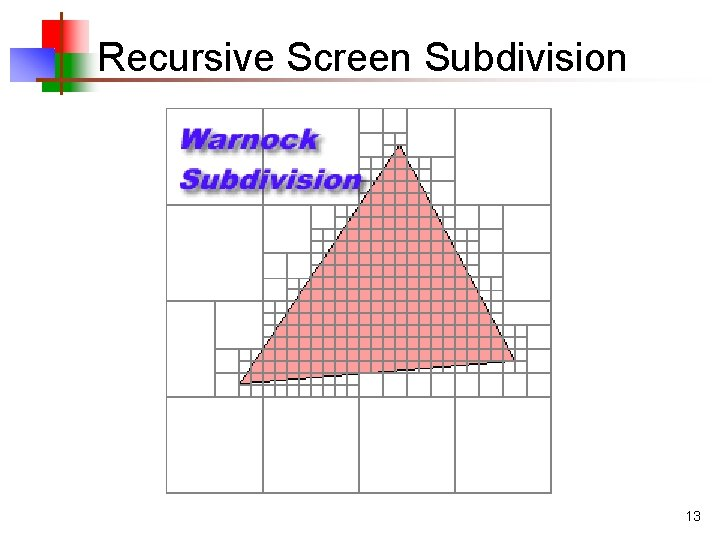Recursive Screen Subdivision 13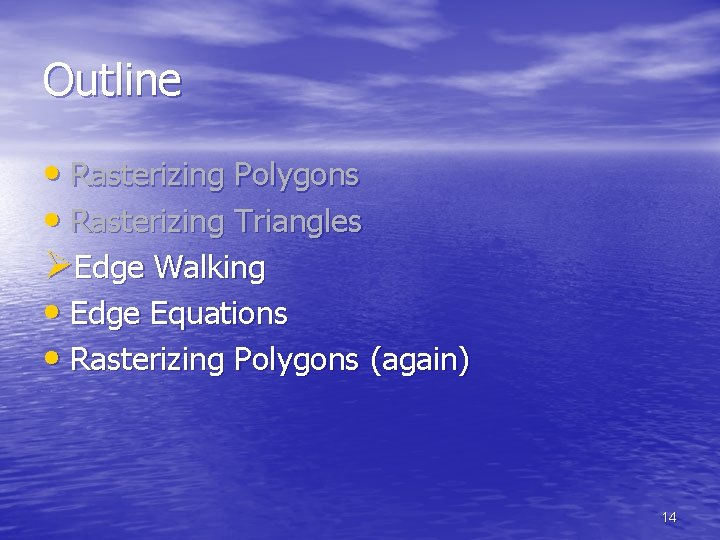Outline • Rasterizing Polygons • Rasterizing Triangles ØEdge Walking • Edge Equations • Rasterizing Polygons (again) 14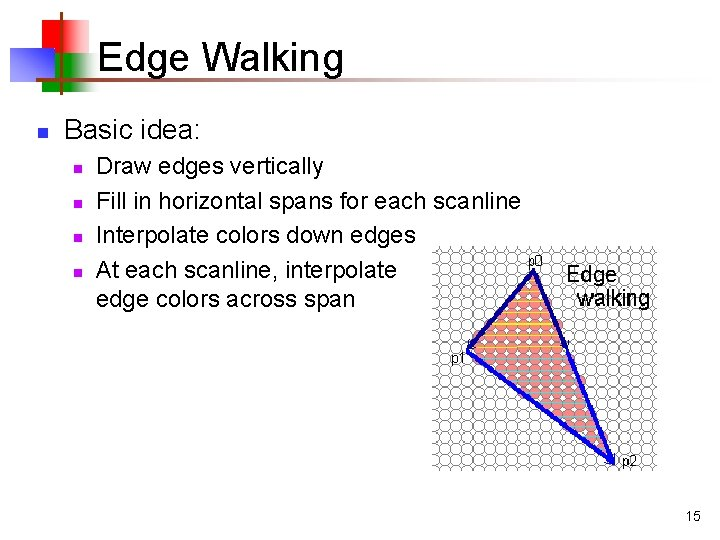Edge Walking n Basic idea: n n Draw edges vertically Fill in horizontal spans for each scanline Interpolate colors down edges At each scanline, interpolate edge colors across span 15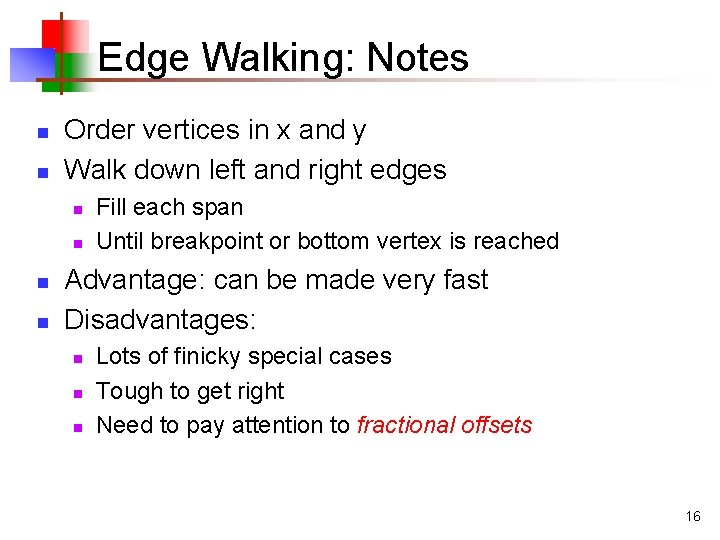Edge Walking: Notes n n Order vertices in x and y Walk down left and right edges n n Fill each span Until breakpoint or bottom vertex is reached Advantage: can be made very fast Disadvantages: n n n Lots of finicky special cases Tough to get right Need to pay attention to fractional offsets 16Edge Walking: Notes n n n Fractional offsets: Be careful when interpolating color values! Also: beware gaps between adjacent edges 17Outline • Rasterizing Polygons • Rasterizing Triangles • Edge Walking ØEdge Equations • Rasterizing Polygons (again) 18Edge Equations n An edge equation is simply the equation of the line containing that edge n n Q: What is the equation of a 2 D line? A: Ax + By + C = 0 Q: Given a point (x, y), what does plugging x & y into this equation tell us? A: Whether the point is: n n n On the line: Ax + By + C = 0 “Above” the line: Ax + By + C > 0 “Below” the line: Ax + By + C < 0 19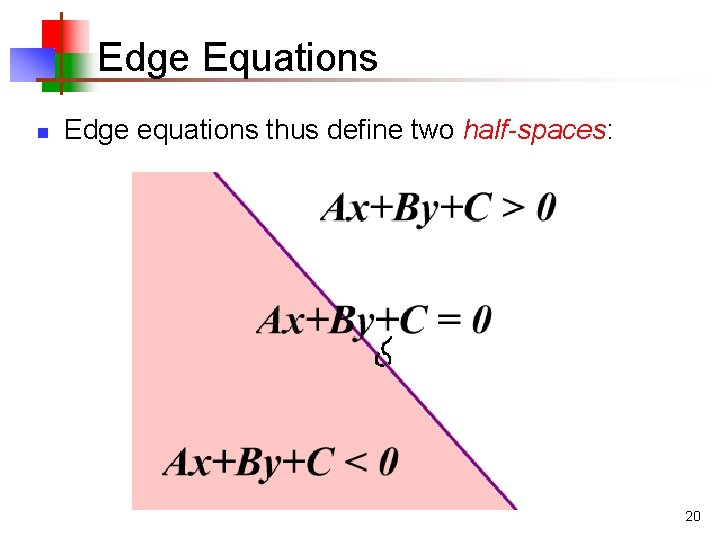Edge Equations n Edge equations thus define two half-spaces: 20Edge Equations And a triangle can be defined as the intersection of three positive half-spaces: +C 3 >0 A 2 x +B +B 2 y 2 y +C +C 2 3 y 3 y +C 3 <0 A 2 x +B +B A 3 x n A 1 x + C 1 + B 1 y A 1 x + 2 <0 >0 >0 C 1 < + y B 1 0 21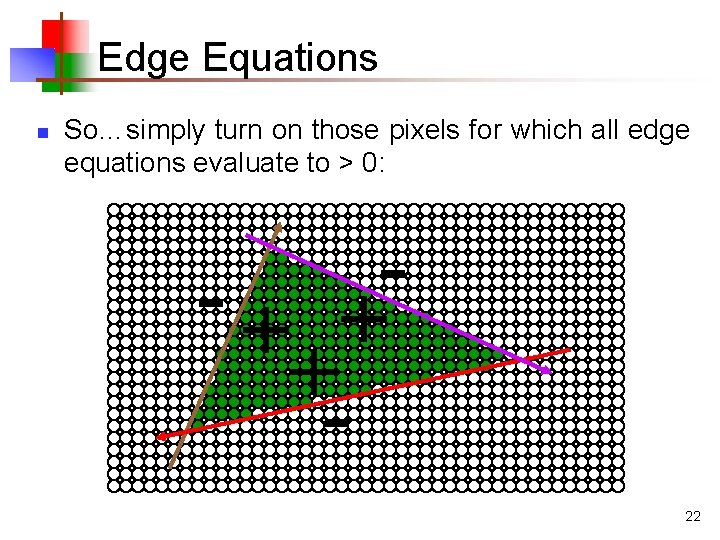Edge Equations n So…simply turn on those pixels for which all edge equations evaluate to > 0: -+ + +22Using Edge Equations n n An aside: How do you suppose edge equations are implemented in hardware? How would you implement an edge-equation rasterizer in software? n n n Which pixels do you consider? How do you compute the edge equations? How do you orient them correctly? 23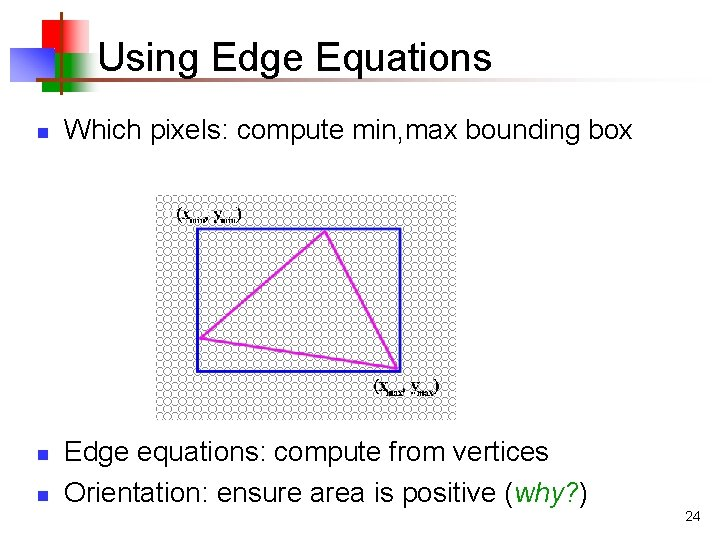Using Edge Equations n n n Which pixels: compute min, max bounding box Edge equations: compute from vertices Orientation: ensure area is positive (why? ) 24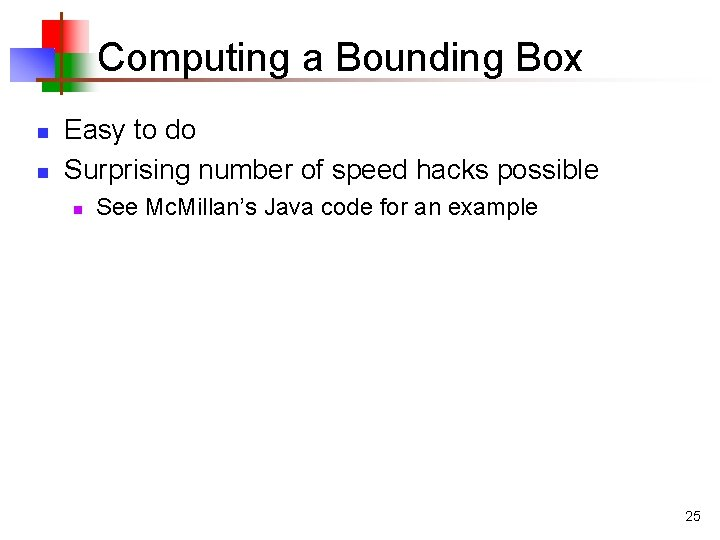Computing a Bounding Box n n Easy to do Surprising number of speed hacks possible n See Mc. Millan’s Java code for an example 25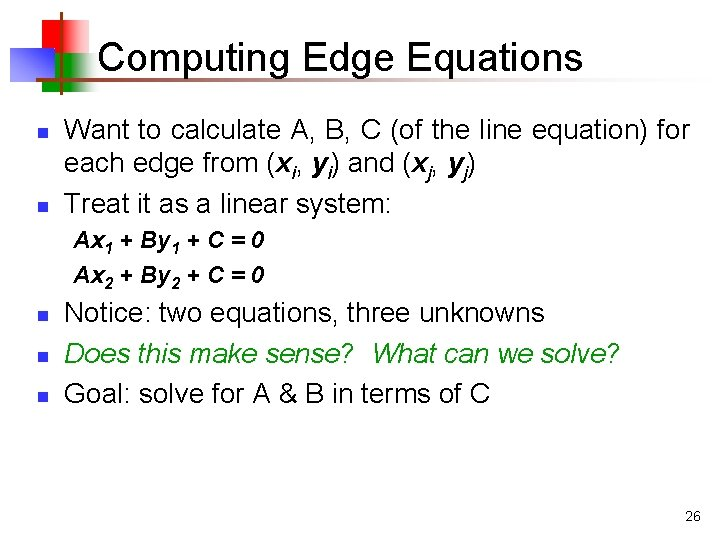Computing Edge Equations n n Want to calculate A, B, C (of the line equation) for each edge from (xi, yi) and (xj, yj) Treat it as a linear system: Ax 1 + By 1 + C = 0 Ax 2 + By 2 + C = 0 n n n Notice: two equations, three unknowns Does this make sense? What can we solve? Goal: solve for A & B in terms of C 26Computing Edge Equations n n n Set up the linear system: Multiply both sides by matrix inverse: Let C = x 0 y 1 - x 1 y 0 for convenience n Then A = y - y and B = x - x 0 1 1 0 27Edge Equations: Numerical Issues n Calculating C = x 0 y 1 - x 1 y 0 involves some numerical precision issues n n When is it bad to subtract two floating-point numbers? A: When they are of similar magnitude n n n Example: 1. 234 x 104 - 1. 233 x 104 = 1. 000 x 101 We lose most of the significant digits in result In general, (x 0, y 0) and (x 1, y 1) (corner vertices of a triangle) are fairly close, so we have a problem 28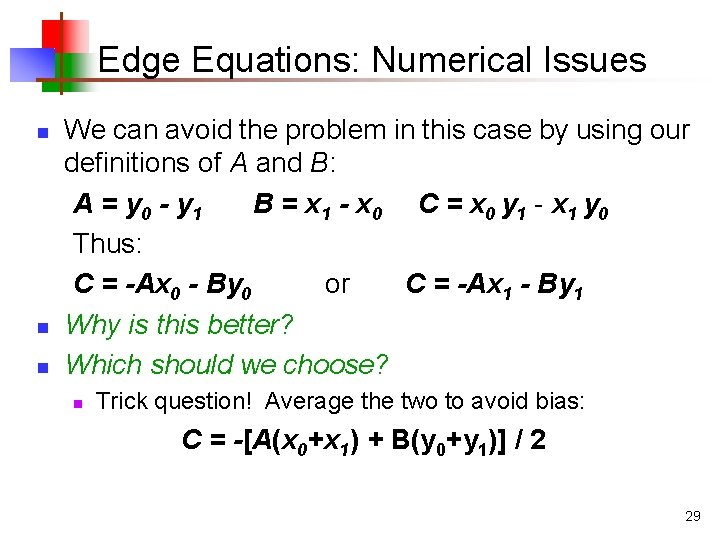Edge Equations: Numerical Issues n n n We can avoid the problem in this case by using our definitions of A and B: A = y 0 - y 1 B = x 1 - x 0 C = x 0 y 1 - x 1 y 0 Thus: C = -Ax 0 - By 0 or C = -Ax 1 - By 1 Why is this better? Which should we choose? n Trick question! Average the two to avoid bias: C = -[A(x 0+x 1) + B(y 0+y 1)] / 2 29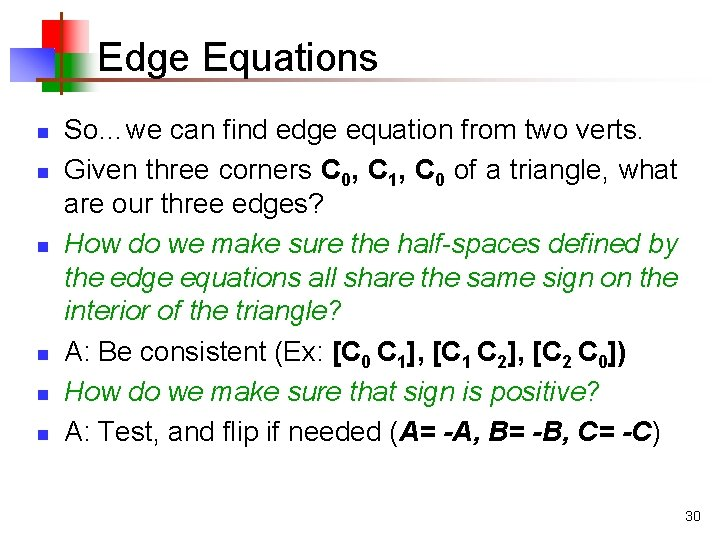Edge Equations n n n So…we can find edge equation from two verts. Given three corners C 0, C 1, C 0 of a triangle, what are our three edges? How do we make sure the half-spaces defined by the edge equations all share the same sign on the interior of the triangle? A: Be consistent (Ex: [C 0 C 1], [C 1 C 2], [C 2 C 0]) How do we make sure that sign is positive? A: Test, and flip if needed (A= -A, B= -B, C= -C) 30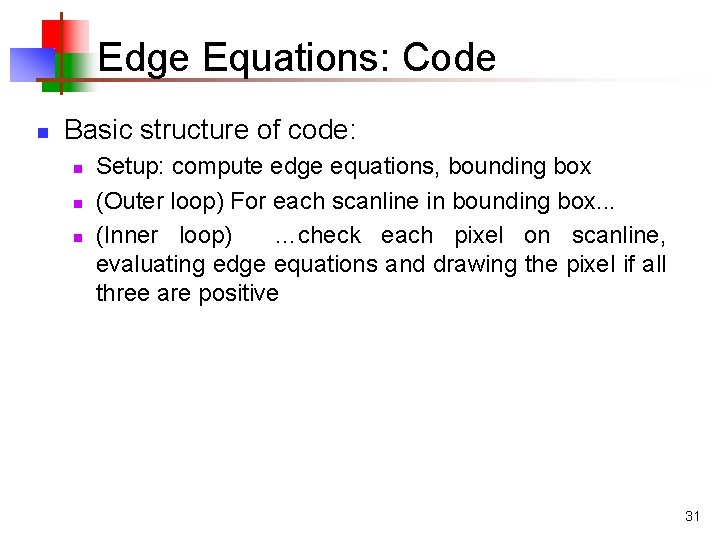Edge Equations: Code n Basic structure of code: n n n Setup: compute edge equations, bounding box (Outer loop) For each scanline in bounding box. . . (Inner loop) …check each pixel on scanline, evaluating edge equations and drawing the pixel if all three are positive 31Optimize This! find. Bounding. Box(&xmin, &xmax, &ymin, &ymax); setup. Edges (&a 0, &b 0, &c 0, &a 1, &b 1, &c 1, &a 2, &b 2, &c 2); /* Optimize this: */ for (int y = y. Min; y <= y. Max; y++) { for (int x = x. Min; x <= x. Max; x++) { float e 0 = a 0*x + b 0*y + c 0; float e 1 = a 1*x + b 1*y + c 1; float e 2 = a 2*x + b 2*y + c 2; if (e 0 > 0 && e 1 > 0 && e 2 > 0) set. Pixel(x, y); } } 32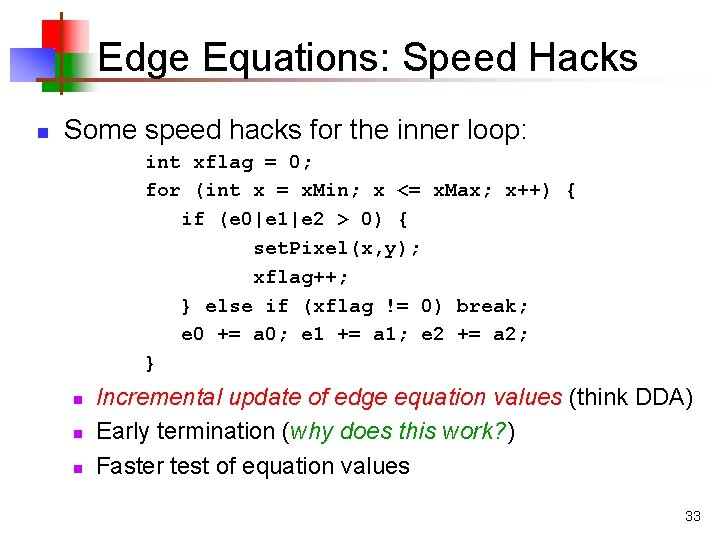Edge Equations: Speed Hacks n Some speed hacks for the inner loop: int xflag = 0; for (int x = x. Min; x <= x. Max; x++) { if (e 0|e 1|e 2 > 0) { set. Pixel(x, y); xflag++; } else if (xflag != 0) break; e 0 += a 0; e 1 += a 1; e 2 += a 2; } n n n Incremental update of edge equation values (think DDA) Early termination (why does this work? ) Faster test of equation values 33Edge Equations: Interpolating Color n n Given colors (and later, other parameters) at the vertices, how to interpolate across? Idea: triangles are planar in any space: n n This is the “redness” parameter space Also need to do this for green and blue Note: plane follows form z = Ax + By + C Look familiar? 34Edge Equations: Interpolating Color n n Given redness at the 3 vertices, set up the linear system of equations: The solution works out to: 35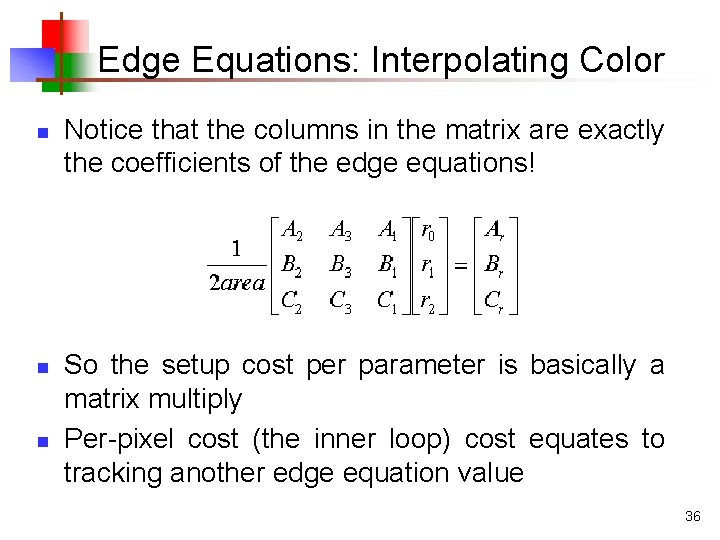Edge Equations: Interpolating Color n n n Notice that the columns in the matrix are exactly the coefficients of the edge equations! So the setup cost per parameter is basically a matrix multiply Per-pixel cost (the inner loop) cost equates to tracking another edge equation value 36Triangle Rasterization Issues n n n Exactly which pixels should be lit? A: Those pixels inside the triangle edges What about pixels exactly on the edge? n n n Draw them: order of triangles matters (it shouldn’t) Don’t draw them: gaps possible between triangles We need a consistent (if arbitrary) rule n Example: draw pixels on left or top edge, but not on right or bottom edge 37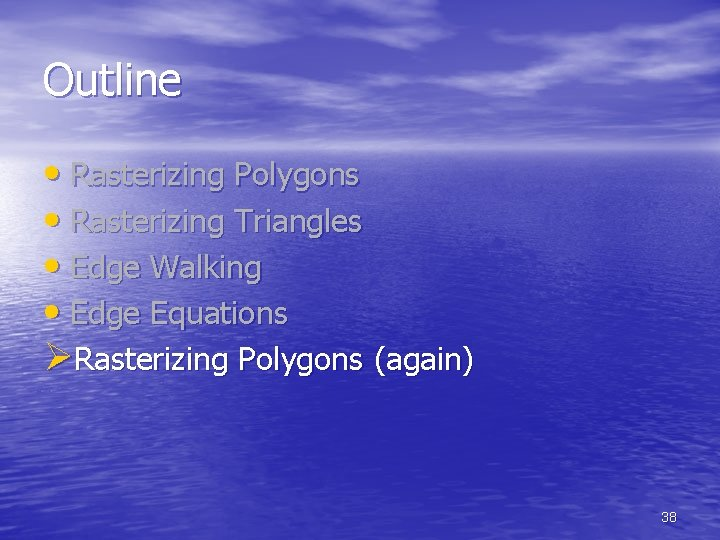Outline • Rasterizing Polygons • Rasterizing Triangles • Edge Walking • Edge Equations ØRasterizing Polygons (again) 38General Polygon Rasterization n n Now that we can rasterize triangles, what about general polygons? We’ll not take an edge-equations approach (why? ) 39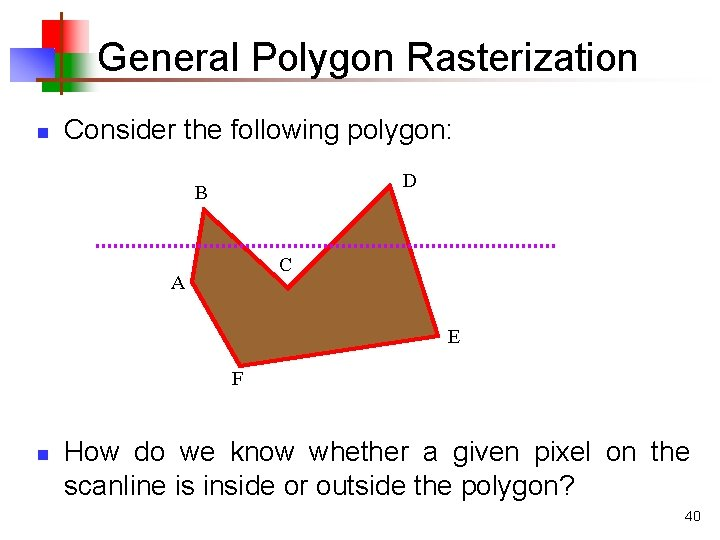General Polygon Rasterization n Consider the following polygon: D B C A E F n How do we know whether a given pixel on the scanline is inside or outside the polygon? 40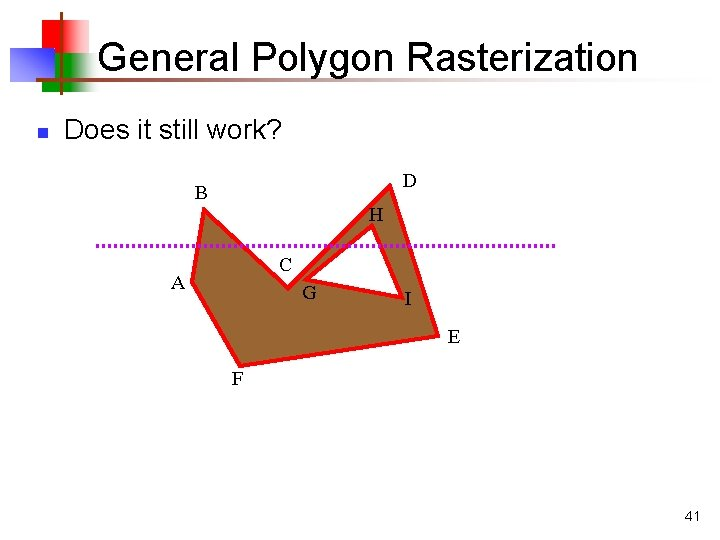General Polygon Rasterization n Does it still work? D B H C A G I E F 41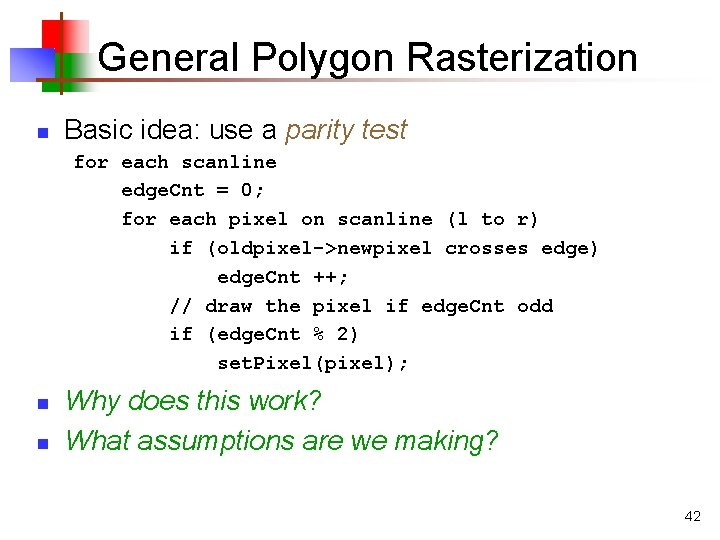General Polygon Rasterization n Basic idea: use a parity test for each scanline edge. Cnt = 0; for each pixel on scanline (l to r) if (oldpixel->newpixel crosses edge) edge. Cnt ++; // draw the pixel if edge. Cnt odd if (edge. Cnt % 2) set. Pixel(pixel); n n Why does this work? What assumptions are we making? 42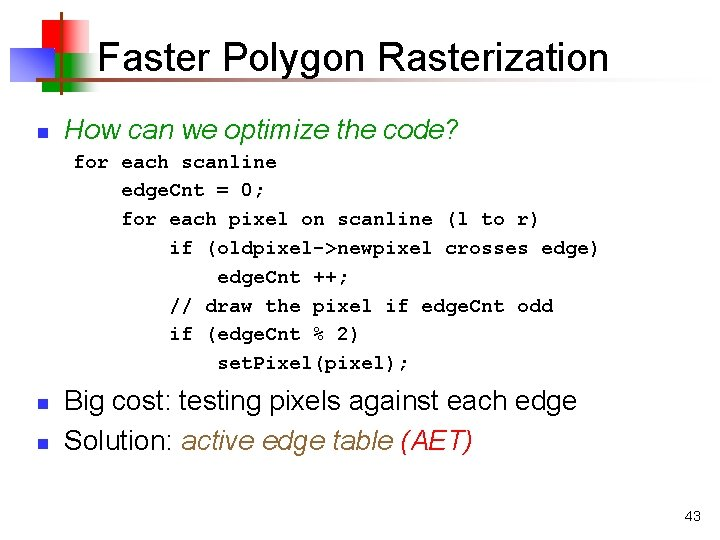Faster Polygon Rasterization n How can we optimize the code? for each scanline edge. Cnt = 0; for each pixel on scanline (l to r) if (oldpixel->newpixel crosses edge) edge. Cnt ++; // draw the pixel if edge. Cnt odd if (edge. Cnt % 2) set. Pixel(pixel); n n Big cost: testing pixels against each edge Solution: active edge table (AET) 43Active Edge Table n Idea: n n Edges intersecting a given scanline are likely to intersect the next scanline Within a scanline, the order of edge intersections doesn’t change much from scanline to scanline 44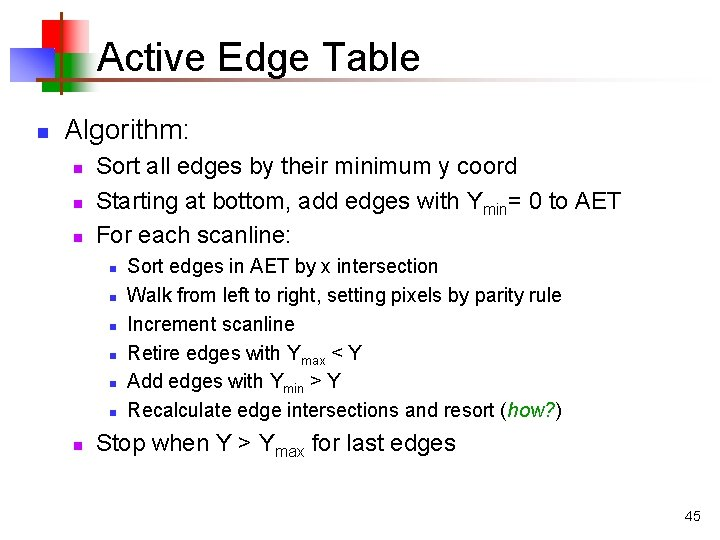Active Edge Table n Algorithm: n n n Sort all edges by their minimum y coord Starting at bottom, add edges with Ymin= 0 to AET For each scanline: n n n n Sort edges in AET by x intersection Walk from left to right, setting pixels by parity rule Increment scanline Retire edges with Ymax < Y Add edges with Ymin > Y Recalculate edge intersections and resort (how? ) Stop when Y > Ymax for last edges 45